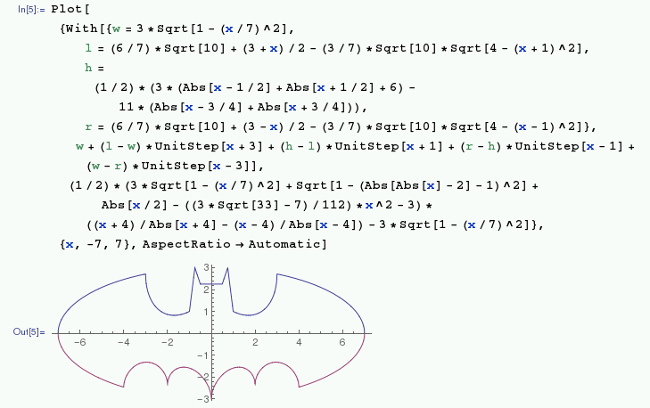# Do advanced math with Mathematica on the Raspberry Pi

## Wolfram bundles a version of Mathematica with Raspbian. Learn how to use it in the 12th article in our series on getting started with Raspberry Pi.Image credits :
João Trindade. Modified by Jason Baker. CC BY-SA 2.0.

## Subscribe now

Get the highlights in your inbox every week.

In the mid-'90s, I started college as a math major, and, even though I graduated with a computer science degree, I had taken enough classes to graduate with a minor—and only two classes short of a double-major—in math. At the time, I was introduced to an application called Mathematica by Wolfram, where we would take many of our algebraic and differential equations from the blackboard into the computer. I spent a few hours a month in the lab learning the Wolfram Language and solving integrals and such on Mathematica.

Mathematica was closed source and expensive for a college student, so it was a nice surprise to see almost 20 years later Wolfram bundling a version of Mathematica with Raspbian and the Raspberry Pi. If you decide to use another Debian-based distribution, you can download it on your Pi. Note that this version is free for non-commercial use only.

The Raspberry Pi Foundation's introduction to Mathematica covers some basic concepts such as variables and loops, solving some math problems, creating graphs, doing linear algebra, and even interacting with the GPIO pins through the application.

## raspberrypi_12_mathematica_batman-plot.pngTo dive deeper into Mathematica, check out the Wolfram Language documentation. If you just want to solve some basic calculus problems, check out its functions. And read this tutorial if you want to plot some 2D and 3D graphs.

Or, if you want to stick with open source tools while doing math, check out the command-line tools expr, factor, and bc. (Remember to use the man command to read up on these utilities.) And if you want to graph something, Gnuplot is a great option.